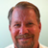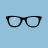# Mulltiple time frames question

O

##### New member
I have assembled the following code to check for MACD crossing on the 15min chart and plotting a signal line on the 5 min. It results in three lines plotting. One for each 5 min candle. My question, is it possible to display only one signal at the last 5 min candle in the 15 min range. Any help is appreciated.

Code:
``````script MACD15 {
input TimeFrame = 5;
def agg = TimeFrame * 60000;
def vClose15 = close(period = agg);

input fastLength = 12;
input slowLength = 26;
input MACDLength = 9;
input averageType = AverageType.EXPONENTIAL;

DEF Value = MovingAverage(averageType, vClose15, fastLength) - MovingAverage(averageType, vClose15, slowLength);

DEF Avg = MovingAverage(averageType, Value, MACDLength);

DEF STRONG_BULL_MACD = if Value crosses above Avg and Value < 0 then 1 else 0;

PLOT STRONG_BEAR_MACD = if Value crosses below Avg and Value > 0 then 1 else 0;

}

input fastLength = 12;
input slowLength = 26;
input MACDLength = 9;
input averageType = AverageType.EXPONENTIAL;

DEF Value = MovingAverage(averageType, CLOSE, fastLength) - MovingAverage(averageType, CLOSE, slowLength);

DEF Avg = MovingAverage(averageType, Value, MACDLength);

DEF STRONG_BULL_MACD = if Value crosses above Avg and Value < 0 then 1 else 0;

DEF STRONG_BEAR_MACD = if Value crosses below Avg and Value > 0 then 1 else 0;

plot BULL_CONVERGENCE = IF MACD15(15).STRONG_BULL_MACD then 1 else 0;General question about strategies Questions 1Heiken Ashi Pivot "Strategy" - mobius - help question. Questions 2